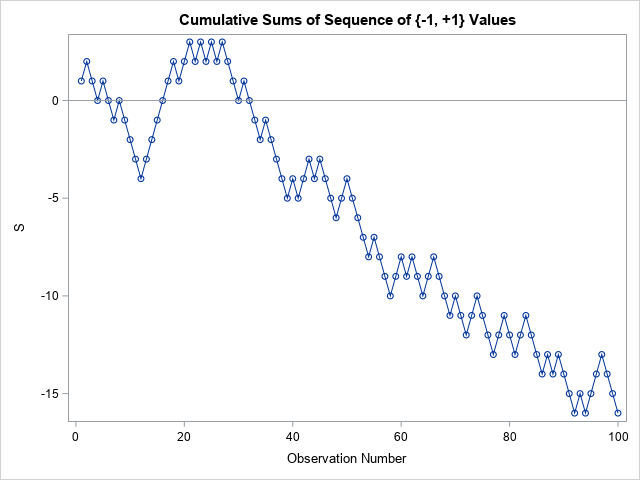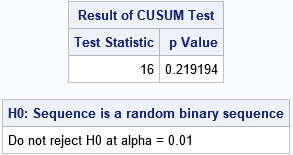Many statistical tests use a CUSUM statistic as part of the test. It can be confusing when a researcher refers to "the CUSUM test" without providing details about exactly which CUSUM test is being used. This article describes a CUSUM test for the randomness of a binary sequence. You start with a long sequence of binary values such as heads and tails from a coin toss. The test tries to determine whether the sample comes from a Bernoulli distribution with probability p=0.5. In short, is the binary sequence random?

The CUSUM test for randomness of a binary sequence is one of the NIST tests for verifying that a random or pseudorandom generator is generating bits that are indistinguishable from truly random bits (Rukin, et al., 2000, revised 2010, pp 2-31 through 2-33). The test is straightforward to implement. You first translate the data to {-1, +1} values. You then compute the cumulative sums of the sequence. If the sequence is random, the cumulative sum is equivalent to the position of a random walker who takes unit steps. If the sequence is random, the sums will not move away from 0 (the expected sum) too quickly. I've previously visualized the random walk with unit steps (sometimes called a "Drunkard's walk").

Before proceeding to the CUSUM test, I should mention that this test is often used in conjunction with other tests, such as the "runs test" for randomness. That is because a perfectly alternating sequence such as 0101010101... will pass the CUSUM test even though the sequence is clearly not randomly generated. In fact, any sequence that repeatedly has k zeros followed by k ones also passes the test, provided that k is small enough.

### The CUSUM test for randomness of a binary sequence in SAS

The NIST report contains an example of calling the CUSUM test with a sequence of length N=100. The following SAS/IML statements define a sequence of {0, 1} values, convert those values to {-1, +1}, and plot the cumulative sums:

```proc iml; eps = {1 1 0 0 1 0 0 1 0 0 0 0 1 1 1 1 1 1 0 1 1 0 1 0 1 0 1 0 0 0 1 0 0 0 1 0 0 0 0 1 0 1 1 0 1 0 0 0 1 1 0 0 0 0 1 0 0 0 1 1 0 1 0 0 1 1 0 0 0 1 0 0 1 1 0 0 0 1 1 0 0 1 1 0 0 0 1 0 1 0 0 0 1 0 1 1 1 0 0 0 }; x = 2*eps - 1; /* convert to {-1, +1} sequence */ S = cusum(x);   title "Cumulative Sums of Sequence of {-1, +1} Values"; call series(1:ncol(S), S) option="markers" other="refline 0 / axis=y" label="Observation Number";```The sequence contains 58 values of one category and 42 values of the other. For a binomial distribution with p=0.5, the probability of a sample that has proportions at least this extreme is about 13%, as shown by the computation 2*cdf("Binomial", 42, 0.5, 100);. Consequently, the proportions are not unduly alarming. However, to test whether the sequence is random, you need to consider not only the proportion of values, but also the sequence. The graph of the cumulative sums of the {-1, +1} sequence shows a drift away from the line S=0, but it is not clear from the graph whether the deviation is more extreme than would be expected for a random sequence of this length.

The CUSUM test gives you a way to quantify whether the sequence is likely to have occurred as a random draw from a Bernoulli(p=0.5) distribution. The test statistic is the maximum deviation from 0. As you can see from the graph, the test statistic for this sequence is 16. The NIST paper provides a formula for the probability that a statistic at least this extreme occurs in a random sequence of length N=100. I implemented a (vectorized) version of the formula in SAS/IML.

```/* NIST CUSUM test for randomness in a binary {-1, +1} sequence. https://nvlpubs.nist.gov/nistpubs/legacy/sp/nistspecialpublication800-22r1a.pdf Section 2.13. Pages 2-31 through 2-33. INPUT: x is sequence of {-1, +1} values. */ start BinaryCUSUMTest(x, PrintTable=1, alpha=0.01); S = colvec( cusum(x) ); /* cumulative sums */ n = nrow(S); z = max(abs(S)); /* test statistic = maximum deviation */   /* compute probability of this test statistic for a sequence of this length */ zn = z/sqrt(n); kStart = int( (-n/z +1)/4 ); kEnd = int( ( n/z -1)/4 ); k = kStart:kEnd; sum1 = sum( cdf("Normal", (4*k+1)*zn) - cdf("Normal", (4*k-1)*zn) );   kStart = int( (-n/z -3)/4 ); k = kStart:kEnd; sum2 = sum( cdf("Normal", (4*k+3)*zn) - cdf("Normal", (4*k+1)*zn) ); pValue = 1 - sum1 + sum2;   /* optional: print the test results in a nice human-readable format */ cusumTest = z || pValue; if PrintTable then do; print cusumTest[L="Result of CUSUM Test" c={"Test Statistic" "p Value"}]; labl= "H0: Sequence is a random binary sequence"; if pValue <= alpha then msg = "Reject H0 at alpha = " + char(alpha); /* sequence seems random */ else msg = "Do not reject H0 at alpha = " + char(alpha); /* sequence does not seem random */ print msg[L=labl]; end; return ( cusumTest ); finish;   /* call the function for the {-1, +1} sequence */ cusumTest = BinaryCUSUMTest(x);```According to the CUSUM test, there is not sufficient evidence to doubt that the sequence was generated from a random Bernoulli process.